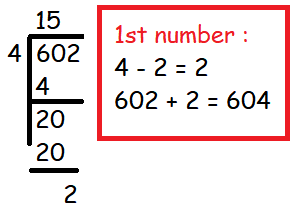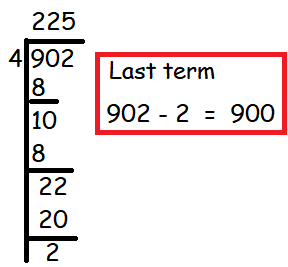### Find the sum of all natural numbers between 602 and 902 which are not divisible by 4.

Find the sum of all natural numbers between 602 and 902 which are not divisible by 4.
Solution :
Sum of all natural number between 602 and 902
=  Sum of all number between 602 and 902 - Sum of numbers which are divisible by 4 between 602 and 902
Sum of all number between 602 and 902 :
=  [603 + 604 +................ + 901]
 n = [(l - a)/d] + 1 n = [(901-603)/1]+1 n = 298 + 1 n  = 299 Sn  = (n/2)[a + l] S301  = (299/2)[603 + 901]   =  (299/2)   =  299(752)   =  224848
Sum of numbers which are divisible by 4 between 602 and 902.=  604 + 608 + 612 + .......... + 900
n  = [(l - a)/d] + 1
=  [(900 - 604)/4] + 1
n  = 75
Sn  = (n/2)[a + l]
S75  = (75/2)[604 + 900]
=  (75/2)
=  75(752)
=  56400
Required sum  = 224848 - 56400
=  168448